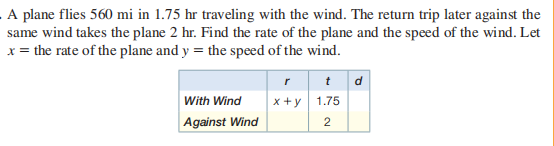### ¿Todavía tienes preguntas de matemáticas?

Pregunte a nuestros tutores expertos
Algebra
PreguntaA plane flies $$560 mi$$ in $$1.75 hr$$ traveling with the wind. The return trip later against the same wind takes the plane $$2 hr$$ . Find the rate of the plane and the speed of the wind. Let $$x =$$ the rate of the plane and $$y =$$ the speed of the wind.

$$\text { plane: } 300 mph ; \text { wind: } 20 mph$$